Project tutorial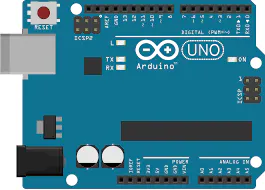# Simple UNO calculator © GPL3+

Make simple math with arduino UNO

• 28,016 views
• 26 respects

## Components and suppliesArduino UNO Just the board will do it, no need for more. Also, if 'im not mistaken, this will work with any board, original or not. If it works with Arduino IDE, this project works on it.
×1Arduino 101
×1Arduino Nano R3
×1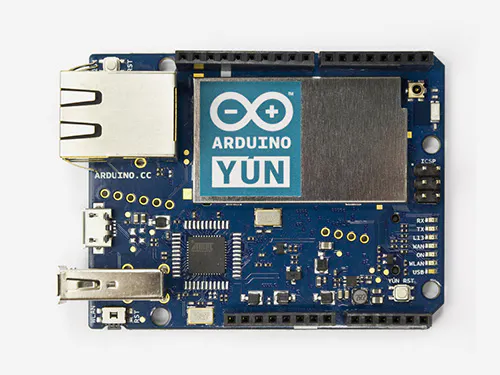Arduino Yun
×1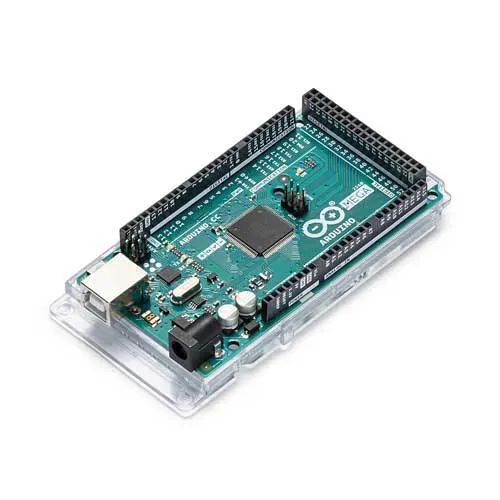Arduino Mega 2560
×1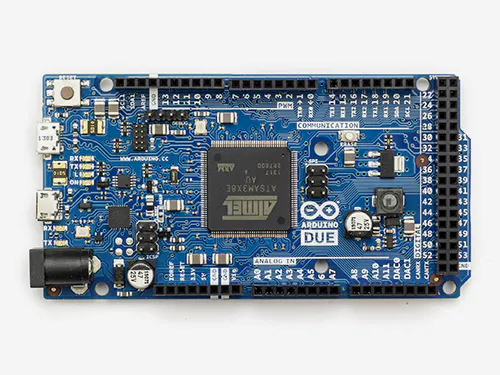Arduino Due
×1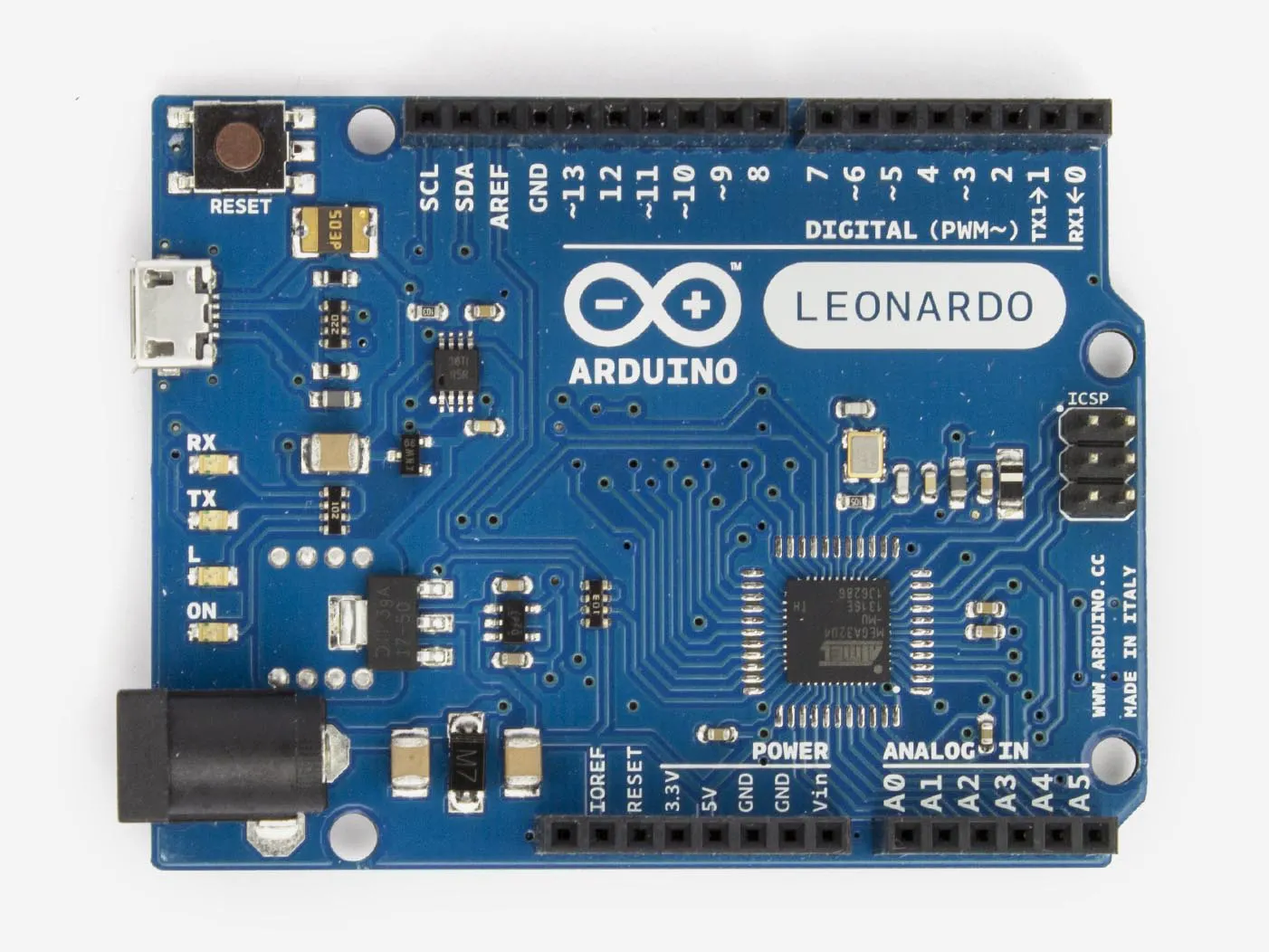Arduino Leonardo
×1

## Apps and online services

One day, my math teacher asked me as I was talking about arduino : "Can you do math with it?".I replied "I don't know", and that got me thinking about how to make that happen. Then, I kinda figured it out how to do it and i wanna share now.

So here it is! A very simple way of doing math with arduino. For my project, I used arduino UNO, but I belive it works with any of them.

The step-by-step is very simple:

• Connect the board with the computer
• Enable Serial monitor
• Write a calculation that is valid (See the code for more instructions)

I plan to make a better calculator, but for now it's just that : a way of doing basic math. If you want the "better" calculator, please let me know.

Stay tuned to see a better "calculator"! Hope to see you soon!

## Code

##### CODE FOR CALCULATORArduino
You enter the numbers and the signal togheter, like "2+3", or 8*2, and it gives the result
```/* Serial arduino calculator
in this project, you can make basic
arithmetic with the help of arduino,
almost like a very rustic calculator.
It accepts two numbers and a signal, and
makes the operation, witch can be of +, -, * or /.
E.G. : send "2+3" (Without quotes and with no
space separing the info), and arduino answers 5.
Digit "7-3" and arduino te responde com 4.
Criado por João Paulo Rodrigues Poltronieri

This code is on public domain
*/

// first of all, create variables to store
// the information sent to arduino

lng number1; // first number of the calculation,
// sent through the Serial monitor

// If you take a look, it's a long varible, so
// we're able to use big numbers

long number2; // second number sent through the SM

char calSignal; // create a char variable to store
// the calcuation signal.

long result; // result of the calculation

void setup() {
Serial.begin(9600); // begins serial communications
Serial.println("Send me a calculation");
Serial.println("E.G. : 2+3");
Serial.println();
// prints this to test serial communication, and
// prints a line space
}

void loop() {
while(Serial.available() > 0) {
// while there are dada being sent to arduino,

number1 = Serial.parseInt();
// number1 will be the first number

// Note the use of "Serial.parseInt, so,
// in case you use 23, it stores in
// number1 the number 23

// if we used Serial.read(), it would
// only store 2

calSignal = Serial.read(); // calSignal will be the first
// info after the first number

number2 = Serial.parseInt(); // stores the second
// number in number2

resolucao(); // Custom function to solve the calculations

Serial.println(result);
// Prints the result of the calculation
Serial.println(); // jumps a line
Serial.println("Outra conta, por favor"); // prints
Serial.println(); // jumps a line
}
}

void resolucao() { // Custom function that
// solves the calculations

switch (calSignal) {
// Here we use "switch...case" to save some space on
// the sketch. It's, basicaly, a function that verifies
// various "if" statements.

// Here, it verifies what's the value held by
// calSigna. Basicaly, it verifies the "signal"
// of the calculation

case '+' : // if calSignal is '+'
result = number1 + number2; // sums the numbers
// and makes result hold the value of the calculation
break; // break to exit the "case"
case '+' : // if calSignal is '+'
result = number1 - number2; // subtracts the numbers
// and makes result hold the value of the calculation
break; // break to exit the "case"
case '+' : // if calSignal is '+'
result = number1 * number2; // multiplies the numbers
// and makes result hold the value of the calculation
break; // break to exit the "case"
case '/' : // se calSignal for '/'
result = number1 / number2; // divides the numbers
// and makes result hold the value of the calculation
// PS: in case the division isn't exact, the result
// will be the nearest integrer
break; // break to exit the "case"
default : // If it's not any of these...
Serial.println("CONTA INVÁVIDA");
// Creates an "error"
Serial.println();
}
}
```

## Schematics

Basicaly, just the board is needed, actually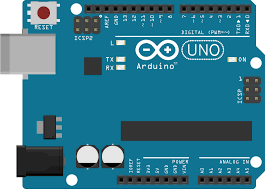#### Published on

September 17, 2017

#### Members who respect this project

See similar projects
you might like

#### 3-Bit Binary Calculator Using Arduino Uno

Project showcase by 22warehamD

• 11,517 views
• 17 respects

#### Simple Arduino Uno - ESP 8266 Integration

Project tutorial by circuito.io team

• 133,574 views
• 110 respects

#### Simple LCD Timer With Arduino UNO

Project showcase by Hugo Blanc

• 64,662 views
• 42 respects

• 3,444 views
• 1 comment
• 9 respects

#### Simple ATTiny13 Shield for Arduino UNO

Project showcase by Hugo Blanc

• 2,920 views